## Introduction

Considerable effort has been devoted to developing mechanical resonators based on low-dimensional materials, such as carbon nanotubes1,2,3,4,5,6,7,8,9,10,11,12, semiconducting nanowires13,14,15,16,17,18,19,20,21,22, graphene23,24,25,26,27,28,29 and monolayer semiconductors30,31,32. The specificity of these resonators is their small size and their ultra-low mass, which enables sensing of force and mass with unprecedented sensitivities7,10. Such high-precision sensing capabilities hold promise for studying physical phenomena in new regimes that have not been explored thus far, for instance, in spin physics33, quantum electron transport34,35, light-matter interaction19 and surface science36,37. However, the transduction of the mechanical vibrations of nanoscale mechanical systems into a measurable electrical or optical output signal is challenging. As a result, force and mass sensing is often limited by the imprecision in the measurement of the vibrations, and cannot reach the fundamental limit imposed by thermo-mechanical noise.

A powerful method to obtain efficient electrical readout of small resonators is to amplify the interaction between mechanical vibrations and the readout field using a superconducting microwave cavity27,28,29. Increasing the field in the cavity improves the readout sensitivity and eventually leads to dynamical back-action on the thermo-mechanical noise. This effect has been studied intensively on comparatively large micro-fabricated resonators, resulting for instance in enhanced optomechanical damping38,39, ground-state cooling of mechanical vibrations40,41 and displacement imprecision below the standard quantum limit42,43. Another phenomenon often observed when detecting and manipulating the motion of mechanical resonators is the induced heating that can occur through Joule dissipation and optical adsorption28,44. Heating is especially prominent in tiny mechanical resonators because of their small heat capacity. An additional difficulty in characterizing mechanical vibrations is related to the fluctuations of the mechanical resonant frequency, also called frequency noise, which are particularly sizable in small resonators endowed with high-quality factors Q10.

Here we study the force sensitivity of multilayer graphene mechanical resonators coupled to superconducting cavities. In particular, we quantify how the force sensitivity is affected by dynamical back-action, Joule heating and frequency noise upon increasing the number of pump photons inside the cavity. We demonstrate a force sensitivity of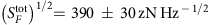, of which ≈50% arises from thermo-mechanical noise and ≈50% from measurement imprecision. The force sensitivity tends to be limited by measurement imprecision and frequency noise at low pump power, and by optomechanical damping and Joule heating at high pump power.

## Results

### Thermal force noise and imprecision force noise

A fundamental limit of force sensing is set by the thermo-mechanical noise of the eigenmode that is measured. According to the fluctuation-dissipation theorem, the associated thermal force noise is white and is quantified bywhere Tmode is the temperature of the mechanical eigenmode, and meff is its effective mass8,45. This force noise is transduced into a mechanical resonance with line widthand height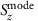in the displacement spectrum (Fig. 1). Importantly, equation (1) shows that the low mass of graphene decreases the size of the thermo-mechanical force noise. However, a drawback of tiny resonators with high Q-factors is their tendency to feature sizable frequency noise that broadens the resonance and, therefore, increases the size of the force noise10,46.

Measuring mechanical vibrations with high accuracy is key to resolving small forces, since the imprecision in the measurement contributes to the force sensitivity. The force sensitivity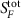is given by the sum of the thermal force noiseand the imprecision force noise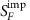, where the latter is the result of the white noise background with strength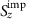in the displacement spectrum (Fig. 1a). The challenge with mechanical resonators based on low-dimensional systems is to reach the limit. When detecting the motion of graphene resonators with microwave cavities, one typically operates in the resolved sideband limit27,28,29, where the cavity decay rate κ is significantly smaller than the mechanical resonance frequency ωm. This is interesting for force sensing, because pumping on the red sideband allows to enhance the mechanical damping rate by Γopt, and therefore to reduce the harmful effect of frequency noise, as we will discuss below. In addition, this allows to increase the measurement bandwidth, as is often done in magnetic resonance force microscopy experiments33 while keeping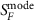constant. The drawback of red sideband pumping compared to pumping at the cavity resonant frequency is an increased imprecision force noise at high pump powers. In the red-detuned pump regime, the measurement imprecision contributes to the force sensitivity by the amount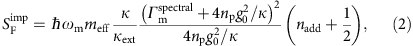with κext the external coupling rate of the cavity, nadd the noise added by the amplifier chain at the output of the device, Γmspectral the intrinsic line width of the resonator, np the number of pump photons in the cavity, and g0 the single-photon optomechanical coupling. Figure 1b shows the pump power dependence of the force sensitivity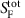expected in the absence of Joule heating and frequency noise. The increase ofat high np is due to the dynamical back-action, which enhances the mechanical line width by Γopt=4npg02/κ.

### Device characterization

Our devices consist of a suspended graphene mechanical resonator capacitively coupled to a superconducting niobium (Nb) cavity (Fig. 2a–c). The graphene resonators are circular with a radius of R≈1.6 μm. Here we present data of two devices. The graphene resonator of device A has a thickness of approximately 25 layers, and the one of device B 5–6 layers. This corresponds respectively to an effective mass of meff=(4.1±0.8)·10−17 kg and (9.6±0.8)·10−18 kg. The uncertainty results from extracting the mass with different methods including optical contrast measurements, thickness measurements with atomic force microscopy and the measured electrostatic softening of the mechanical resonators (see Supplementary Note 1 and Supplementary Equation 2). The fundamental mode of devices A and B vibrates at ωm/2π=67 MHz and ωm/2π=46 MHz at Vg=0 V, respectively. Here Vg is the constant voltage applied between the graphene flake and the superconducting cavity. In order to improve the attachment of the graphene flake to its support, we clamp it between cross-linked poly(methyl metracylate) and the contact electrodes; the detailed fabrication is described elsewhere29. The separation between the graphene resonator and the cavity counter electrode at Vg=0 V is assumed to be equal to the hole depth, which is typically d0≈85 nm in our devices as measured with atomic force microscopy. Varying Vg allows us to tune the separation between the graphene resonator and the cavity counter electrode24,29,47,48,49, modifying the graphene-cavity capacitance, the cavity frequency ωc and ωm (Fig. 2g,h). The superconducting cavity is a coplanar waveguide resonating at about ωc/2π=7.4 GHz. We choose a single-port, quarter wavelength, reflection geometry, so that the cavity is connected to ground on one end, allowing to apply a well-defined constant voltage between the cavity and the graphene flake. The other end of the cavity is coupled to a transmission line via a capacitor Cext with a coupling rate κext=2π × 850 kHz for device A; the total cavity decay rate is κ=κext+κint=2π × 1.8 MHz (see Methods). Here κint accounts for the internal energy loss.

We detect the vibrations of the graphene resonator with high precision by pumping the cavity with an electromagnetic field, and probing its mechanical sideband. This sideband is generated by the capacitive modulation of the pump field at frequency ωp/2π by the graphene vibrations at ωm/2π. We usually set ωp=ωcωm and probe the electromagnetic field that exits the cavity at ωc. We measure the device at the cryostat base temperature of 15 mK if not stated otherwise. The cavity output field is amplified with a high electron-mobility-transistor mounted at the 3 K stage of the cryostat. Mechanical noise spectra are detected with a spectrum analyser at room temperature. For a detailed description of the measurement set-up, see Supplementary Fig. 1 and Supplementary Note 2. In addition, we perform ring-down measurements to determine the mechanical dissipation rate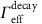of the graphene resonator. Spectral measurements are not suitable for quantifying reliablybecause of the potentially substantial frequency noise of graphene resonators.

We characterize the single-photon optomechanical coupling and show that the coupling can be significantly enhanced by deflecting the membrane towards the cavity electrode. For this, we quantify the optomechanical scattering rate Γopt using ring-down measurements at Vg=0 V and Vg=3.002 V for device A. Figure 3a,b shows the measured dissipation rateas a function of cavity pump photon number np for blue and red detuned pumping. The measurements are well described bywherecorresponds to the intrinsic mechanical dissipation rate, and ± to red and blue detuned pumping at ωp=ωcωm, respectively. By increasing Vg from 0 to 3.002 V we obtain a strong increase of the optomechanical coupling from g0=2π × 9.7 Hz to g0=2π × 42.6 Hz. We estimate that the separation d between the membrane and the cavity counter electrode is reduced from 88 to 33 nm when varying Vg from 0 to 3.002 V. The calibration of both g0 and np is robust, while the quantification of the reduction of d is approximative; see Supplementary Notes 1 and 3, Supplementary Fig. 2 and Supplementary equations (1), (3)–(5).Figure 3: Effective mechanical energy decay rates extracted from ring-down measurements.

### Thermal calibration and sideband cooling

In order to calibrate the mechanical phonon occupation and the mode temperature Tmode, we measure the mechanical thermal motion spectrum while varying the cryostat temperature40. This is done by pumping the cavity with a weak pump tone on the red sideband. The integrated area of the thermal resonance is proportional to the mode temperature according to the equipartition theorem. For temperatures above 100 mK the area is linearly proportional to the cryostat temperature, showing that the mode is in thermal equilibrium with the cryostat (Fig. 4b). This linear dependence serves as a precise calibration to relate the resonance area to the averaged phonon occupation nm and the mode temperature Tmode. Below 100 mK the mechanical mode does not thermalize well with the cryostat. The origin of this poor thermalization at low temperature may be related to the heating induced by the pump field (see below)28, and a non-thermal force noise50 such as the electrostatic force noise related to the voltage noise in the device. As a next characterization step, we investigate the mechanical phonon occupation when increasing the power of the pump tone on the red sideband and keeping the temperature of the cryostat constant at Tcryo=15 mK. The measured resonance gets broader and its area smaller (Fig. 4c), showing that the mechanical mode is damped and cooled38,39. At the largest available pump power, the phonon occupation reaches nm=7.2±0.2 (Fig. 4e). This is the lowest phonon occupation reached in a mechanical resonator based on graphene27,28,51. The error in the estimation of nm is given by the standard error obtained from five successive spectral measurements.Figure 4: Thermal calibration and sideband cooling of fundamental mechanical mode with red-detuned pumping.

### Displacement sensitivity and force sensitivity

The improved coupling allows us to achieve also an excellent displacement sensitivity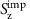(Fig. 4d). At the largest pump power, we obtain, which compares favourably to previous works27,51,52. The error inis given by the uncertainty in the estimation of meff. We obtainfrom the noise floor of the measured power spectral density SN usingwiththe zero-point motion amplitude27. The displacement sensitivity scales as 1/np (Fig. 4d). By comparing the measurement to the expected displacement sensitivity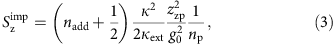we obtain that the equivalent noise added by the amplifier chain is nadd=32. This is a reasonable value for a high electron-mobility-transistor amplifier mounted at 3 K42,53.

We now quantify the force sensitivity as a function of the microwave pump power (Fig. 5a,e). Since the mechanical resonances in the measured displacement spectra are well described by Lorentzian line shapes, the thermal force noise is quantified usingwith the effective mechanical susceptibility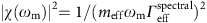. Similarly, we obtain the imprecision force noise with. The best force sensitivity we achieve for device A is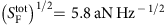with a mechanical bandwidth of 20 kHz (Fig. 5a,d). In device B we reach a force sensitivity ofwith a mechanical bandwidth of 0.2 kHz (see Fig. 5e,h). The error in the estimation of the force sensitivity is obtained from both the uncertainty in the mass and the fluctuations in the measurement of, which we evaluate by calculating the standard error of 10 measurements. This force sensitivity compares favourably with the best sensitivities obtained with micro-fabricated resonators ()42,45, albeit it is not as good as that of resonators based on carbon nanotubes8,10. Compared to previous devices, the mechanical bandwidth of graphene resonators is much higher, which enables faster detection of sudden force changes.

## Discussion

We plot bothandas a function of cavity pump photon population in Fig. 5b. As expected, the imprecision force noise decreases at low np and increases at high np due to the enhanced damping caused by the optomechanical back-action. The thermal force noiseappears roughly constant when varying np as a result of the competing effects of Joule heating and frequency noise. Joule heating is caused by the microwave current in the graphene flake induced by the pump field. This results in the increase of the temperature Tbath of the thermal bath coupled to the mechanical mode as well as the mechanical dissipation rate26,28. We can infer the productfrom the measurements of nm andin Figs 3b and 4e usingWhen increasing the pump power, Joule heating significantly increases the product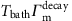(Fig. 5c), and therefore the size of the thermal force noise (equation (1)). We see next that the effect of frequency noise leads to the opposite dependence of the thermal force noise on pump power. Frequency noise enhances the spectral line width by the amount δ Γnoise,when the fluctuations of the resonant frequency are described by a white noise8. The measurements of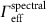andas a function of pump power can be well described by equation (5) with δ Γnoise/2π=8.7 kHz (Fig. 5d). Importantly, Fig. 5d shows thatis comparable toat large pump power, showing that the relative contribution of δ Γnoise togets negligible upon increasing np. As the cooling efficiency described by equation (4) remains unaltered by frequency noise (see chapter 7 in54), the thermal force noise is quantified byTaking into account the measured effects of Joule heating and frequency noise in equation (6), the thermal force noiseis expected to remain roughly constant as a function of np (dark yellow line in Fig. 5b), in agreement with the measurements. Overall, the best force sensitivity we achieve in this device isat np≈4·106 (Fig. 5a). While the force sensitivity in this device is primarily limited by the measurement imprecision, the thermal force noise is affected to a large extent by frequency noise at low np and by Joule heating at high np.

In device B, the graphene resonator has a lower mass and a narrower mechanical line width, two assets for high force sensitivity (Fig. 5e–h). The spectral line width corresponds to a mechanical quality factor of Q≈200,000. In this device we reach a force sensitivity ofat np≈4·105 (see Fig. 5e). In an attempt to improve the thermal anchoring of device B compared to device A, the graphene contact electrodes contain an additional Au layer between the graphene and the Nb layer28,55. The normal metal layer is expected to increase the thermal conductance between the graphene flake and the contact electrodes through electron diffusion, which allows for better heat dissipation into the contacts. However, device B is still strongly affected by Joule heating, which substantially increases the value of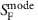when increasing the pump power (Fig. 5f,g). The heating is so strong that we are not able to reduce the phonon occupation nm with sideband cooling. We attribute the strong heating to the fact that the resonator is significantly thinner than the one of device A and therefore has a smaller heat capacity. The effect of frequency noise on the spectral line width is negligible for pump powers above np≈4·105. We do not know the origin of the frequency noise but it might be related to charged two-level fluctuators in the device. The force sensitivity is here primarily limited by the measurement imprecision at low np, and by the thermo-mechanical force noise and Joule heating at high np.

In the future, the force sensitivity of graphene optomechanical devices can be further improved using a quantum-limited Josephson parametric amplifier53. This readout will improve the measurement imprecision, by lowering nadd in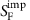. In addition, it will be possible to resolve the thermal vibrations with lower pump power, which is crucial to reduce Joule heating while working with low-mass graphene resonators. A quantum-limited amplifier with nadd=0.5 may allow to achieve 47 zN Hz−1/2 force sensitivity at 15 mK taking the mass of a single-layer graphene resonator with the diameter and the quality factor of device B (Fig. 1b). With only modest device improvements, it may be possible to probe the fundamental limit of continuous displacement detection imposed by quantum mechanics, since the force noise associated to quantum backaction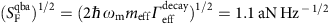is approachingmeasured at np=1.4 × 107 for device A. Force sensing with resonators based on two-dimensional materials hold promise for detecting electron and nuclear spins33 using superconducting cavities compatible with relatively large magnetic fields56, and studying the thermodynamic properties of two-dimensional materials, such as the quantum capacitance and the magnetization35.

## Methods

### Cavity characterization

In Fig. 2d,e we plot the coefficient |S11|2 and the phase of the reflected signal when sweeping the frequency over the cavity resonance at ωc/2π=7.416 GHz. To extract the external coupling rate κext and the internal loss rate κint we fit the measurement with the line shape expected for a one-port reflection cavity57which yields κint/2π=950 kHz and κext/2π=850 kHz at Vg=3.002 V for device A. The rates of Device B are κint/2π=800 kHz and κext/2π=1700 kHz at Vg=0 V.

### Data availability

The data that support the findings of this study are available from the corresponding author upon request.